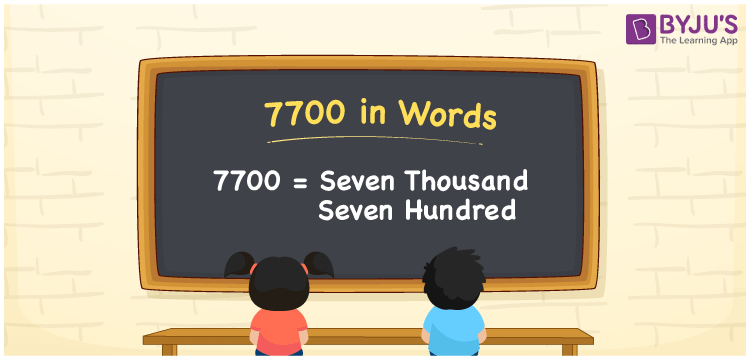# 7700 in Words

7700 in words is Seven thousand seven hundred. The number name of 7700 is expressed as seven thousand seven hundred in both the Indian and International System of Numerals. Hence, converting the number 7700 to words, the place value chart plays a major role. For converting the number 7700 into words, the place value system plays a major role. In this article, we are going to learn the spelling of 7700 in English words with a complete explanation.

 7700 in Words: Seven Thousand Seven Hundred. Seven Thousand Seven Hundred in Numerical Form: 7700.

## 7700 in English Words## How to Write 7700 in Words?

The place value chart will be employed to write the number 7700 in words. The following is the place value table for the number 7700.

 Thousands Hundreds Tens Ones 7 7 0 0

The expanded form of 7700 is as follows:

= 7 × Thousand + 7 × Hundred + 0 × Ten + 0 × One

= 7 × 1000 + 7 × 100

= 7000 + 700

= 7700

= Seven thousand seven hundred

Thus, 7700 in words is seven thousand seven hundred.

7700 in words – Seven thousand seven hundred

Is 7700 an odd number? – No

Is 7700 an even number? – Yes

Is 7700 a perfect square number? – No

Is 7700 a perfect cube number? – No

Is 7700 a prime number? – No

Is 7700 a composite number? – Yes

## Frequently Asked Questions on 7700 in Words

Q1

### Write 7700 in words.

7700 in words is seven thousand seven hundred.

Q2

### Simplify 8000 – 300, and express in words.

Simplifying 8000 – 300, we get 7700. Hence, 7700 in words is seven thousand seven hundred.

Q3

### Is 7700 an even number?

Yes, 7700 is an even number.